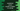# 2 different JavaScript programs to count the number of digits in a string## Write a JavaScript program to count the number of digits in a string:

This post will show you how to find the total number of digits in a string in JavaScript. We will write one program, that will count the total number of digits in a given string.

This problem can be solved in multiple ways. We can simply use one loop to loop through the characters of the string and check if any character is a digit or not.

Another way is by using a regex. Using regex or regular expression, we can filter out the numbers from a string. It returns one array of numbers. We can join them as a string and return the length of the string.

I will show you both of these ways to solve it.

### Method 1: Count the number of digits in a string using for loop:

The idea is to iterate through the characters of a string one by one and check if any character is digit or not. If any character is found as digit, we are incrementing one count value.

``````function findTotalCount(str) {
let count = 0;

for (let ch of str) {
if (ch >= "0" && ch <= "9") {
count++;
}
}
return count;
}

const str1 = "Hello4 World65 123 !!";
const str2 = "123and 456 and 78-1";
const str3 = "Hello World !!";

console.log(findTotalCount(str1));
console.log(findTotalCount(str2));
console.log(findTotalCount(str3));``````

#### Explanation :

In this example,

• str1, str2 and str3 are three given strings.
• findTotalCount function is used to find the total count of digits in a string str that we are passing as its parameter.
• We are initializing one variable count as 0 to hold the total count.
• Using one for…of loop, we are iterating through the chraracters of the string str one by one.
• For each character, we are checking if it a number of not. If yes, we are incrementing the value of count by 1.
• Finally, we are returning count.

#### Output:

The above program prints the below output:

``````6
9
0``````

### Method 2: Using regex to count the number of digits in a string:

In this method, we are using regex to find out the number of digits in a string. Below is the complete program:

``````function findTotalCount(str) {
let digitsArr = str.match(/\d+/g);
if (digitsArr) {
return digitsArr.join("").length;
}
return 0;
}

const str1 = "Hello4 World65 123 !!";
const str2 = "123and 456 and 78-1";
const str3 = "Hello World !!";

console.log(findTotalCount(str1));
console.log(findTotalCount(str2));
console.log(findTotalCount(str3));``````

In this example, we are using one regular expression or regex to get only the numbers from a string. match() method returns one array of numbers. Using join(""), we are joining all values to a single string and returning its length i.e. total numbers found using the regex.

If you run the above example, it will print similar result as the previous one.